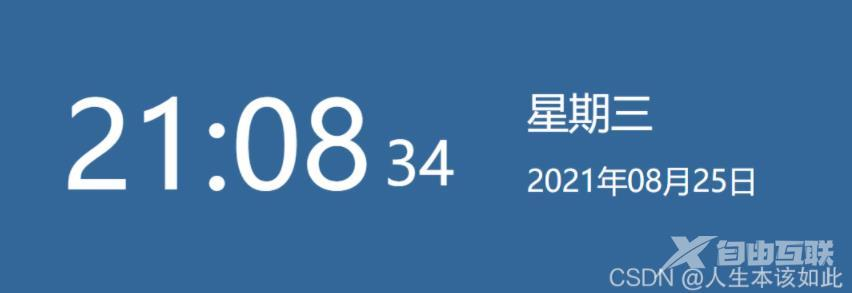﻿ JavaScript实现网页电子时钟 - 自由资讯

# JavaScript实现网页电子时钟```<div class="wrapper">
<div class="time-box">
<div class="hour"></div>
<div class="sec"></div>
</div>

<div class="date">
<div class="week"></div>
<div class="date-box"></div>
</div>
</div>```

```var hour = document.querySelector('.hour');
var sec = document.querySelector('.sec');
var week = document.querySelector('.week');
var dateBox = document.querySelector('.date-box');

//因为定时器第一次会延时一秒，所以第一次会有一秒延迟，提前调用一次
time();

//设置定时器，每秒一次
setInterval(time, 1000)

//获取当地时间，封装成函数
function time() {
var date = new Date();
var y = date.getFullYear();
var m = date.getMonth() + 1;
var d = date.getDate();
var h = date.getHours();
var min = date.getMinutes();
var s = date.getSeconds();
var w = date.getDay();

//补0
m = m < 10 ? '0' + m : m;
d = d < 10 ? '0' + d : d;
h = h < 10 ? '0' + h : h;
min = min < 10 ? '0' + min : min;
s = s < 10 ? '0' + s : s;

hour.innerHTML = h + ':' + m;
sec.innerHTML = s;
week.innerHTML = '星期' + ['一', '二', '三', '四', '五', '六', '日'][w - 1];
dateBox.innerHTML = y + '年' + m + '月' + d + '日';

}```

﻿